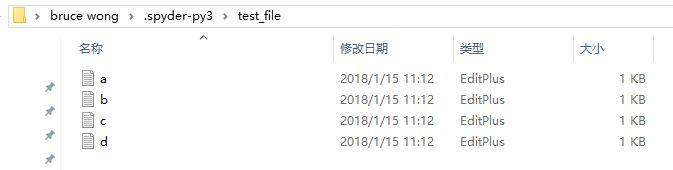2016-05-16 14:42:01 kellyseeme 阅读数 3749
• ###### Python-数据库

Python数据库编程视频教程，数据库是MySQL，讲解Python链接MySQL数据库，并对数据库进行增删改查操作。

10783 人正在学习 去看看 尹成

```import os.path
import time
while os.path.getsize('messages') <1000000000:
f = open('messages','a')
f.write('this is a file/n')
f.close()

print 'file create complted'```

```#22s
start_time = time.time()
f = open('messages','r')
for i in f:
end_time = time.time()
print end_time - start_time
break
f.close()

#22s
start_time = time.time()
f = open('messages','r')
end_time = time.time()
print end_time - start_time
break
f.close()

start_time = time.time()
f = open('messages','r')
f.close()
end_time = time.time()
print end_time - start_time```

python批量读写大数据文件 相关内容

2017-05-17 20:40:59 whgyxy 阅读数 1459
• ###### Python-数据库

Python数据库编程视频教程，数据库是MySQL，讲解Python链接MySQL数据库，并对数据库进行增删改查操作。

10783 人正在学习 去看看 尹成

## python普通文件读写

`open(file, mode='r', buffering=-1, encoding=None, errors=None, newline=None, closefd=True, opener=None)`

‘r’ 只读模式（默认）
‘w’ 只写模式
‘a’ 追加写模式
‘b’ 二进制模式
‘t’ 文本模式（默认）
‘+’ 更新模式（一般和其他模式并用）

### 读写函数

``````f = open(yourfilepath, 'r')

line = line.strip("\n")  # 处理的时候一般要去掉换行符（这里是\n）

pass
f.close()  # 文件用完要记得关闭，可以用with关键字，不用手动关闭，程序会自动关闭

# 以下均用with来读写文件
with open('yourfilepath', 'w') as tmpf:
a = 100; b = 97.5; c = 'Good'
tmpf.write('number=%d  score=%f  result%s' % (a, b, c))
# 或者直接写入文件内容——字符串（或二进制数据）
ss = 'yourstring'
f.write(ss)  # 直接写入

ss = 'yourstring'
f.writeline(ss)  # 写入时会自动加入换行符

ss = ['a', 'b', 'c']
f.writelines(ss)  # 参数为字符串序列``````

## python大文件读写

### 内置方法

open函数返回的其实还是一个生成器，不管文件多大，可以一行一行读取

``````with open(yourfilepath,'r') as f:
for line in f:
pass  # 对每行做处理``````

### pandas

``````### 分批加载训练集
chunksize = 10000
pass  # 对每行做处理

# 或者使用iterator获得迭代对象
loop = True
while loop:
try:
pass  # 对每行做处理
except StopIteration:
loop = False``````

### 分块生成批量数据

``````import numpy as np
import pandas as pd

# batch_size为皮尺寸
# datapath为数据集路径
# labelpath标签路径
def data_iter(batch_size, datapath, labelpath):
while(1):   # 死循环，因为数据要循环读取，epoch的缘故
nb_batch = np.ceil(train_x.shape * 1.0 / batch_size)
for i in range(int(nb_batch)):
start = i*batch_size
end = min((i+1)*batch_size, train_x.shape)
# 生成器生成需要的数据(X,Y)
yield (train_x[start:end], train_y[start:end])``````

python批量读写大数据文件 相关内容

2017-06-27 15:15:12 meiguopai1 阅读数 3518
• ###### Python-数据库

Python数据库编程视频教程，数据库是MySQL，讲解Python链接MySQL数据库，并对数据库进行增删改查操作。

10783 人正在学习 去看看 尹成

python  从文件读写，mysql批量插入测试数据

```# encoding=utf-8
# 测试数据制造
import pymysql
import random
import time
import string
import csv
import os

conn = pymysql.connect(host='192.168.152.128', port=3306, user='root', passwd='root', db='test')

# 循环生成随机数据到插入到mysql中
# 三个字段，id,name,age
class TEST_INSERT_MYSQL:
def __init__(self, conn):
self.conn = conn

def init_mysql_data(self):
try:
cur = conn.cursor()
cur.execute("drop table if exists student")
sql = "create table if not exists student(id int not null AUTO_INCREMENT PRIMARY KEY ,name char(20),age char(3))"  # 自增长
cur.execute(sql)

print(time.ctime())
for i in range(1, 1000):
random_name = ''.join(random.sample(string.ascii_letters + string.digits, 8))  # 8位随机字符
random_age = ''.join(str(random.randint(10, 120)))  # 10-120随机字符
value = (random_name, random_age)
insert_sql = "insert into  student(name,age) values(%s,%s)"
cur.execute(insert_sql, value)
i += 1
conn.commit()
print(time.ctime())
except:
conn.rollback()
print("Insert fail")
cur.close()
conn.close()
#定义了一个写入函数，从文件读取数据插入数据库
def insert_mysql(self, data):
cur = conn.cursor()
print(time.ctime())
for i in range(1, len(data)):
insert_sql = "insert into  student(name,age) values(%s,%s)"
value = (data[i], data[i])
cur.execute(insert_sql, value)
i += 1
conn.commit()
print(time.ctime())
cur.close()
conn.close()

# 读写操作txt文本文件,定义一个写函数一个读函数
class OPREATE_FILE():
# 写测试文件
def write_file(self, filename):
if os.path.exists(filename):  # 删除存在的测试文件
os.remove(filename)
with open(filename, 'w') as f:
for i in range(1, 100):
random_name = ''.join(random.sample(string.ascii_letters + string.digits, 8))
random_age = str(random.randint(10, 120))
list = [str(i)]
list.append(random_name)
list.append(random_age)
data = ','.join(list)
f.write(data + '\n')
assert isinstance(i, object)
i += i

# 读测试文件
list = []
with open(filename, 'r') as f:
for lines in f:
line = lines.split(',')
data = (line, line, line.replace("\n", ""))
list.append(data)

return list

#以下为测试部分
Test = TEST_INSERT_MYSQL(conn)
# Test.insert_mysql_data()

Test_data = OPREATE_FILE()
# Test_data.write_file('test_data.txt')
Test.insert_mysql(A)
```

python批量读写大数据文件 相关内容

2019-08-19 17:59:42 lchmyhua88 阅读数 155
• ###### Python-数据库

Python数据库编程视频教程，数据库是MySQL，讲解Python链接MySQL数据库，并对数据库进行增删改查操作。

10783 人正在学习 去看看 尹成

python  从文件读写，mysql批量插入数据

``````# -*- coding: utf-8 -*-

import os
import mysql.connector

from sqlalchemy import text
import gzip
import json,datetime,decimal

config = {
'host': '127.0.0.1',
'database': 'testuser',
'raise_on_warnings': True,
}
cnx = mysql.connector.connect(**config)

fname = '/htdocs/python/user_log.txt.zip'
f = gzip.open(fname, "rb")
i = 0
list = []
for line in f:
#strs = line.split("\t")
st = line.decode('utf-8').replace('\n', '')
#if len(st) != 10:
#continue
data = (st,st,st,st,st,st,st.replace("\n", ""))
list.append(data)
cursor = cnx.cursor()
sql = "insert into user_log(id,name,nickname,create_date,uuid,update_date,is_del)values(%s,%s,%s,%s,%s,%s,%s)"
if i > 3:
cursor.executemany(sql, list)
cnx.commit()
print("插入")
i = 0
list.clear()
i = i + 1
if i > 0:
cursor.executemany(sql, list)
cnx.commit()
cnx.close()
f.close()
print("ok")

if __name__ == "__main__":

python批量读写大数据文件 相关内容

2018-08-19 17:17:25 csucsgoat 阅读数 667
• ###### Python-数据库

Python数据库编程视频教程，数据库是MySQL，讲解Python链接MySQL数据库，并对数据库进行增删改查操作。

10783 人正在学习 去看看 尹成

1、创建一个文件夹test_file，里面包含4个文件2、通过os模块读取文件夹的所有文件

``````In : import os
In : os.listdir(r'C:\Users\BruceWong\.spyder-py3\test_file')
Out: ['a.txt', 'b.txt', 'c.txt', 'd.txt']``````
• 1
• 2
• 3

3、读取每个文件内容

``````In : for info in os.listdir(r'C:\Users\BruceWong\.spyder-py3\test_file'):
...:     domain = os.path.abspath(r'C:\Users\BruceWong\.spyder-py3\test_file') #获取文件夹的路径，此处其实没必要这么写，目的是为了熟悉os的文件夹操作
...:     info = os.path.join(domain,info) #将路径与文件名结合起来就是每个文件的完整路径
...:     info = open(info,'r') #读取文件内容
...:     info.close()
...:
...:
aaa
bbb
ccc
ddd``````

python批量读写大数据文件 相关内容